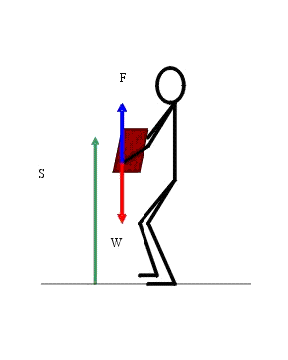See a collection of solved examples for this topic on our website buzztutor.com

# Positive and Negative Work

We have seen the situations when the work done is zero. Work done can also be positive or negative. When 0o <= θ < 90o, work done is positive as  Cos θ is positive. Work done by a force is positive if the applied force has a component in the direction of the displacement. When a body is falling down, the force of gravitation is acting in the downward direction. The displacement is also in the downward direction. Thus the work done by the gravitational force on the body is positive. Consider the same body being lifted in the upward direction. In this case, the force of gravity is acting in the downward direction. But, the displacement of the body is in the upward direction. Since the angle between the force and displacement is 180o, the work done by the gravitational force on the body is negative.Note, that in this case the work done by the applied force which is lifting the body up is positive since the angle between the applied force and displacement is positive.

Thus work done by the  applied force which is lifting up the body is positive since the angle between the applied force and displacement is positive. Thus work done is negative when 90o < θ <= 180o as Cos θ is negative. We can also say that work done by a force is negative if the applied force has a component in a direction opposite to the displacement.

Similarly, frictional force is always opposing the relative motion of the body. When a body is dragged along a rough surface, the frictional force will be acting in the direction opposite to the displacement. The angle between the frictional force and the displacement of the body will be 180o. Thus, the work done by the frictional force will be negative.